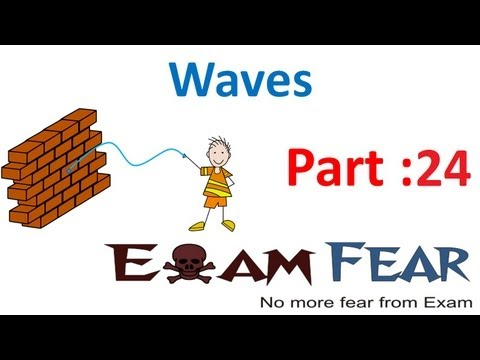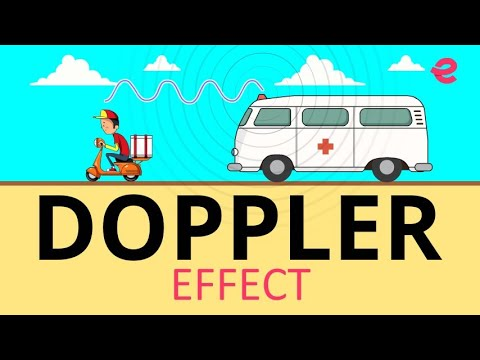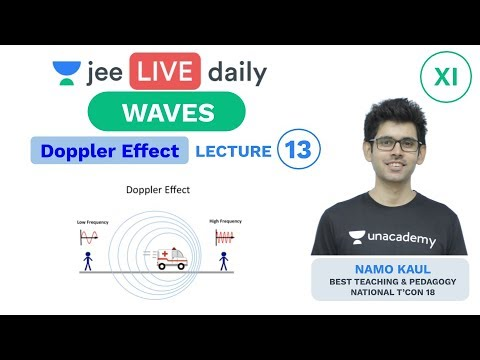Courses

# Test: Doppler Effect

## 10 Questions MCQ Test Physics For JEE | Test: Doppler Effect

Description
This mock test of Test: Doppler Effect for JEE helps you for every JEE entrance exam. This contains 10 Multiple Choice Questions for JEE Test: Doppler Effect (mcq) to study with solutions a complete question bank. The solved questions answers in this Test: Doppler Effect quiz give you a good mix of easy questions and tough questions. JEE students definitely take this Test: Doppler Effect exercise for a better result in the exam. You can find other Test: Doppler Effect extra questions, long questions & short questions for JEE on EduRev as well by searching above.
QUESTION: 1

### In Doppler effect change in frequency depends on

Solution:

The reason for the Doppler effect is that when the source of the waves is moving towards the observer, each successive wave crest is emitted from a position closer to the observer than the crest of the previous wave.

QUESTION: 2

### A sound source of frequency 600 Hz is moving towards an observer with velocity 20m/s. The speed of sound is 340m/s. The frequency heard by observer will be

Solution:

F(s)/F(l) = [V+V(s)]/[V+V(l)]
V = velocity of sound = 340m/s
V(l) = velocity of listener = 0
F(l) = frequency heard by listener = ?
V(s) = velocity of source = -20m/s (because, it's source to listener)
F(s) = frequency of source = 600hz
By putting these values in the above formula and solving we get,
F(l) = 637.5 Hz.
Hence C is correct.

QUESTION: 3

### A radar sends a radio signal of frequency 9 x 109 Hz towards an aircraft approaching the radar. If the reflected wave shows a frequency shift of 3 X 103 Hz, the speed with which the aircraft is approaching the radar in m/s is (velocity of radio signal is 3 X 108 m/s).

Solution:

Given:
Frequency of radio signal n=9×10 9Hz
Frequency shift n0=3×103Hz
Frequency shift shown by reflected wave is Shift =n−n
=>n−n=(v+u/v−u)n−n

Shift=(2u/v−u)n
Now, putting the given values in Eq. (i), we get,
3×103=(2u/3×10-8−u)9×109

⇒3×108−u=2u×9×109/3×103
=6u×106
⇒6×106u+u=3×108

u(6×106+1)=3×108

u(6000001)=3×108

u=3×108/6000001=49.999≈50m/s

QUESTION: 4

A police car moving at 22 m/s, chases a motor cyclist. The police man sounds his horn at 176 Hz while both of them move towards a stationary siren of frequency 165 Hz. Calculate the speed of the motor cyclist, if it is given that he does not hear any beats. (Velocity of sound = 330 m/s).

Solution:

In the first situation, a police car (source of sound) with a speed vs​, is approaching a motorcycle (observer), which is moving away from a police car with a speed of vm​.
Therefore, apparent frequency of sound heard by motorcyclist,

n′=ncar​(​v−vm /v−vs ​​) ...................eq1
.
In second situation, motorcyclist (observer) is approaching a stationary siren (source), with a speed of vm​.
Therefore, apparent frequency of sound heard by motorcyclist,

n′′=nsiren​(v+vm/v​​) ...................eq2 ,.

Now as no beats are observed by motorcyclist, this is possible only when difference in frequencies heard by motorcyclist is zero,
i.e.   n′−n′′=0,
or    n′=n′′,
or    ncar​(​v−vm /v−vs ​​)=nsiren​(v+vm/v​​)
Given n′=176Hz, n′′=165Hz, v=330m/s, vs​=22m/s,ncar​=176Hz  and  nsiren​=165Hz
Hence, 176(v−vm​​/330−22) =165(v+vm/330​​),
or          (​v−vm/ v+vm ​​)=165​/176×308/330​=7/8 ,
or         15vm​=v,
Speed of sound in air v=330 m/s
or         vm​=330/15=22m/s

QUESTION: 5

If a source of sound was moving toward a receiver at 1/3 the speed of sound, what would the resulting wavelength be?

Solution:
QUESTION: 6

Doppler’s effect in sound is:

Solution:

Sound waves require a material medium for their propagation. Therefore we say that the Doppler Effect in sound is asymmetric.

QUESTION: 7

Which medical instrument uses doppler effect?

Solution:

In medicine, the Doppler Effect can be used to measure the direction and speed of blood flow in arteries and veins. This is used in echocardiograms and medical ultrasonography and is an effective tool in diagnosis of vascular problems.

QUESTION: 8

A whistle producing sound waves of frequencies 9500 Hz and above is approaching a stationary person with speed v ms-1. The velocity of sound in air is 300 ms-1. If the person can hear frequencies up to 10000 Hz, the maximum value of v upto which he can the whistle is

Solution:

From Doppler's effect
1000=9500(300/300−v​)⇒v=15m/s

QUESTION: 9

If the source of sound moves at the same speed or faster than speed of the wave then it results in

Solution:

The Doppler Effect is observed whenever the speed of the source is moving slower than the speed of waves. But if the source actually moves at the same speed as or faster than the waves, a different phenomenon is observed. This phenomenon is known as Shock waves or Sonic Booms.

QUESTION: 10

Two trains A and B approach a station from opposite sides, sounding their whistles. A stationary observer on the platform hears no beats. If the velocities of A and B are 15 m/s and 30 m/s respectively and the real frequency of the whistle of B is 600 Hz, the real frequency of the whistle of A is (Velocity of sound = 330 m/s).

Solution:

When no beats is listened at the station thus we get the relative frequencies of both the trains are the same.
Hence the relative frequency of the train B is 600 (330/300) = 660
similarly the relative frequency of train A is f(330/315) = 660
Hence we get f = 630Hz

Track your progress, build streaks, highlight & save important lessons and more!

### Similar Content### Related tests